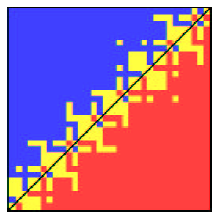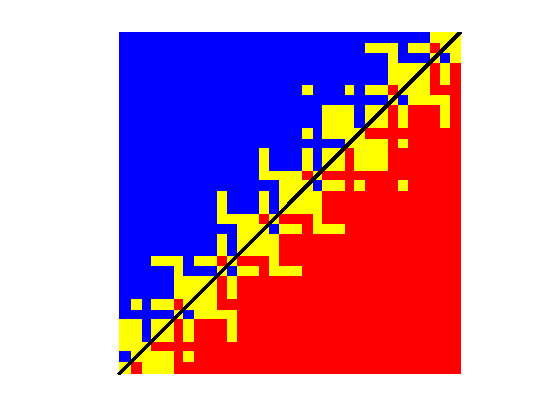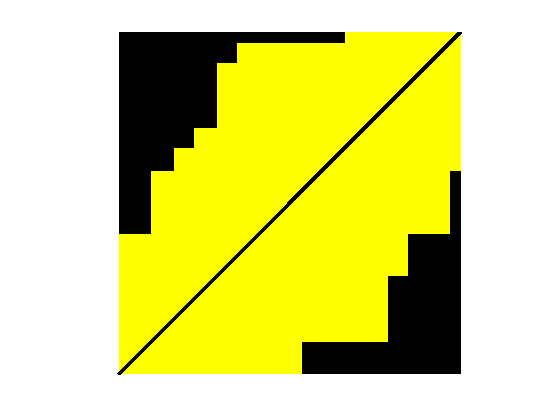# Visualizing the floating-point behavior of the point-on-line test

Today's post is about this picture, which is from the paper "Classroom Examples of Robustness Problems in Geometric Computations," by L. Kettner, K. Mehlhorn, S. Pion, S. Schirra, and C. Yap, Computational Geometry, vol. 40, n. 1, May 2008, pp. 61-78.Yes, I know, I was supposed to do another follow-up on my Cody problem of eliminating unnecessary polygon vertices. But I really am following up on the Cody problem, if a bit indirectly.

I was looking at solution 108897 by Cris, and I paused over these lines:

v1 = p2-p1;
v2 = p3-p2;
if ( v1(1)*v2(2) == v1(2)*v2(1) ) && any(v1.*v2>0)


I recognized the expression ( v1(1)*v2(2) == v1(2)*v2(1) ) as a way to see if the point p2 is on the line segment formed by p1 and p3. It reminded me of Loren's 06-Jun-2008 blog post on methods for determining collinearity, and it also reminded me of the paper above.

I decided to see if I could reproduce the figure in MATLAB.

The figure concerns the behavior of floating-point methods for computing the planar orientation predicate. Given three points $p$, $q$, and $r$ in a plane, does the point $r$ lie to the left of the line through $p$ and $q$, to the right of that line, or on the line? One way to compute this is via the equation

$$O(p,q,r) = \mbox{sign}((q_x - p_x)(r_y - p_y) - (q_y - p_y)(r_x - p_x))$$

The figure in the paper represents an experiment using $q=(12,12)$, $r=(24,24)$, and $256^2$ different locations for the point $p$, all of which are extremely close to the point $p=(0.5,0.5)$. More specifically, the figure is a visualization of $O((p_x+xu, p_y+yu),q,r)$ for $0 \leq x,y \leq 255$ and $u=2^{-53}$. This value of $u$ is the increment between adjacent double-precision floating-point numbers in the interval $0.5 < x \leq 1$.

Here's a straightforward implementation of the orientation equation above.

function out = floatOrient(p,q,r)

out = sign((q(1) - p(1))*(r(2) - p(2)) - ...
(q(2) - p(2))*(r(1) - p(1)));


Let's see what we get when we compute the orientation for all those different point locations $p'$ that are extremely close to $(0.5,0.5)$.

[m,n] = meshgrid(0:255);
Px = 0.5 + n*eps(0.5);
Py = 0.5 + m*eps(0.5);

q = [12 12];
r = [24 24];

out = zeros(size(Px));
for u = 1:256
for v = 1:256
out(u,v) = floatOrient([Px(u,v), Py(u,v)], q, r);
end
end


(Yes, I know ... floatOrient and the calling code above can all be vectorized.)

I'll show in yellow the locations $p'$ where $r$ has been determined to be on the line containing $p'$ and $q$. I'll use blue and red to show where $r$ has been determined to lie on one side or the other of the line.

image(out + 2);
axis equal;
set(gca,'ydir','normal')
axis off
colormap([0 0 1; 1 1 0; 1 0 0])
hold on
plot([.5 256.5],[.5 256.6],'k','LineWidth',3)
hold offHow do we interpret this? First, I want to emphasize that this matrix represents the results for $p'$ that are all extremely close to $(0.5,0.5)$. The lower left corner corresponds exactly to the $(0.5,0.5)$, while the upper right corner corresponds to approximately $(0.500000000000028,0.500000000000028)$. Ideally, only the results lying along the black line should be yellow; these are the locations where $p'$, $q$, and $r$ are collinear. Our floating-point computation method is showing many other locations in yellow. Notice also that a few pairs of locations $p'$ are actually being computed reversed. A left turn has been determined to be a right turn, and vice versa.

In the comment thread on Loren's collinearity post, Tim Davis suggested using a rank test to determine collinearity. It looks something like this:

function out = rankCollinear(p,q,r)
out = rank([p-q ; q-r]) < 2;


Let's see how this test does on our collection of points.

for u = 1:256
for v = 1:256
out(u,v) = rankCollinear([Px(u,v), Py(u,v)], q, r);
end
end

image(out + 1);
axis equal;
set(gca,'ydir','normal')
axis off
colormap([0 0 0; 1 1 0])
hold on
plot([.5 256.5],[.5 256.6],'k','LineWidth',3)
hold offWe only see yellow pixels, not red and blue, because rankCollinear only checks for collinearity, not the left or right turning of noncollinear points. The width of the section of yellow pixels depends on the numerical tolerance used by the rank function.

I am fascinated by this particular experiment and visualization. It reminds me once again that seemingly straightforward geometric ideas can be devilishly difficult to implement with a computer program.

Published with MATLAB® 7.14

|